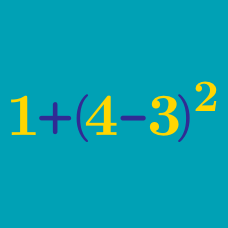Everyday Math

# Basic Arithmetic - Problem Solving

The sum of two numbers is 104 and their difference is 32. What is the value of the larger number?

Evaluate $-2\times(5-2)+\left(2^{2+2}+8^{\frac{2}{3}}\right)^2.$

Historians have just discovered ancient artifacts from the ruins of an old Mesopotamian city. The artifacts included ten bags each containing ten bars of gold. Each bag of gold was created to celebrate every centennial from 1500 B.C. to 600 B.C.. Each gold bar was designed to weigh $12\text{ kg},$ i.e. each bag of gold was designed to contain $120\text{ kg}$ of gold. However, one of these bags consists of gold bars each weighing $10\text{ kg},$ due to the lack of gold in the period that they were made.

Esther the scholar is trying to figure out which bag contains the $10\text{ kg}$-bars of gold. She can take out any number of bars from any bag, but the weighing scale is to be used only once. Esther comes up with a plan: she takes out one bar from the first bag (made in 1500 B.C.), two bars from the second (made in 1400 B.C.), three bars from the third (made in 1300 B.C.), and so on. Thus she will take ten bars from the last bag (made in 600 B.C.). She puts all the bars she picked out using this method on the weighing scale, and the scale reads: $646\text{ kg}.$ In what year was the bag that contains $10\text{ kg}$-bars made?

10 distinct integers from the set $\{1, 2, \ldots, 100\}$ are chosen and their sum is 954. What is the smallest of these 10 numbers?

If the product of two positive numbers is $640$ and the quotient of them is $10,$ what is the sum of the two numbers?

×